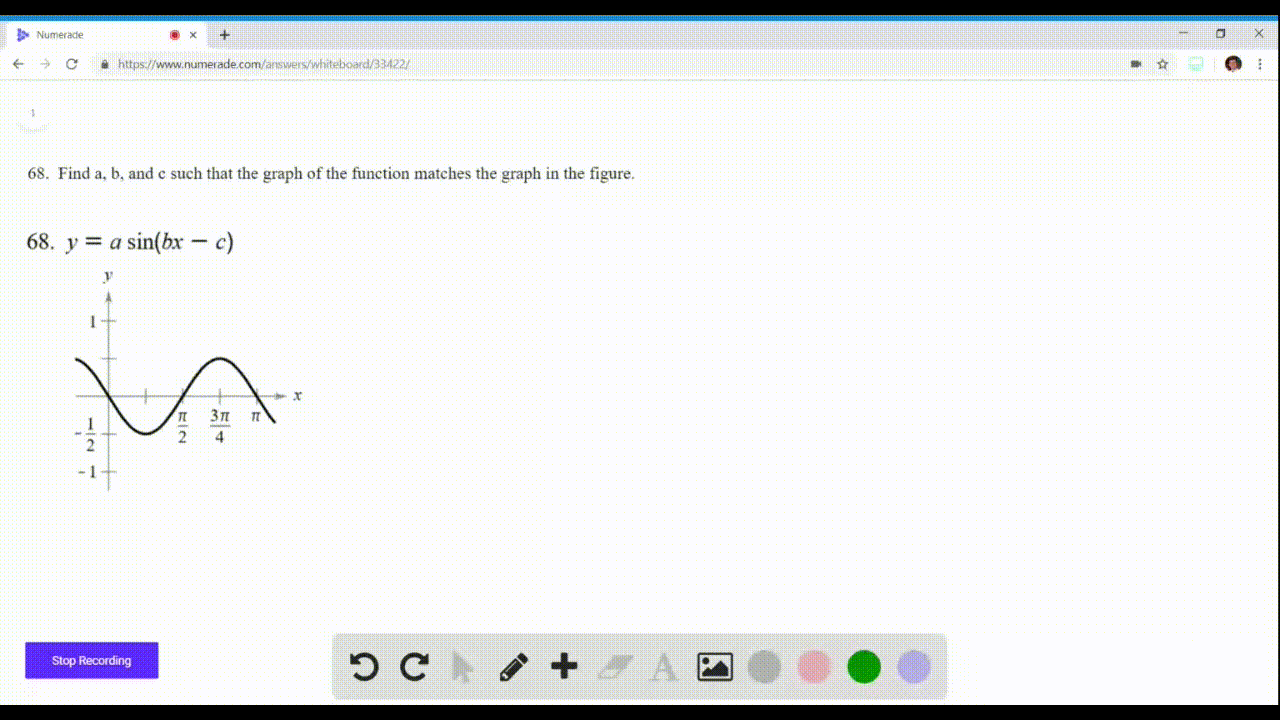🎉 The Study-to-Win Winning Ticket number has been announced! Go to your Tickets dashboard to see if you won! 🎉View Winning Ticket### Graphical Reasoning In Exercises 67 and $68,$ fin…

ViewUniversity of California, Berkeley
Problem 67

# Graphical Reasoning In Exercises 67 and $68,$ find $a, b,$ and $c$ such that the graph of the function matches the graph in the figure.$$y=a \cos (b x-c)$$

## Discussion

You must be signed in to discuss.

## Video Transcript

Okay, so we're asked to write an equation. Why Her phone? Uh, the coefficient terms. Why? This is a co sign of the ex minister. Okay, so we know aids the amplitude, right? So it passes to and it's a little hard lesson for So let's say it's approximately three. So since the attitude is always absolute value of a, um so if we had absolute value of A, that could be either plus minus three. Okay, Now, for part B for another party, I'm looking for BEA, which is our period. What a period of coastline is two pi over feet. And from the looks of this, our period is almost for pie. So it's this secret for pipe. This offer B B would equal 1/2 an important part me our original coast autograph. But it looks like a sign right coast. I'm usually starts, uh, like over here somewhere. So it seems like they shifted it by prior to it. See if you could fire every cube, make a good positive. Great. So our equation is why he could, too. A 123 co sign B, which is 1/2 X minus five bridges